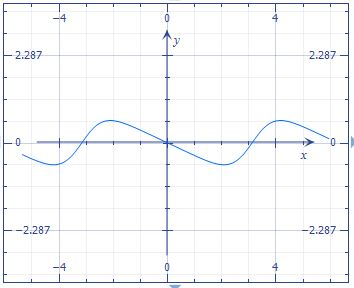# Draw the following function and find its critical points (if any). f(x) = - \frac {\sin x}{2+\cos x}

## Question:

Draw the following function and find its critical points (if any).

{eq}f(x) = - \frac {\sin x}{2+\cos x} {/eq}

## Critical Points:

The critical points of a function can be maximum, minimum or saddle points. There are two ways to classify the critical points, one is with "the first derivative test" and another way is evaluating the critical points in the second derivative of the function, or known as "the second derivative test".

We have the function:

{eq}f(x) = - \frac {\sin x}{2+\cos x} \\ {/eq}

Differentiating the function

{eq}f'(x)=-{\frac {\cos \left( x \right) }{2+\cos \left( x \right) }}-{\frac { \left( \sin \left( x \right) \right) ^{2}}{ \left( 2+\cos \left( x \right) \right) ^{2}}} \\ {/eq}

{eq}f'(x)=0 {/eq} when {eq}x= \frac{8}{3} \pi \\ x=- \frac{8}{3} \pi \\ x=-\frac{2}{3} \pi \\ x=\frac{2}{3} \pi \\ x=\frac{4}{3} \pi \\ x=- \frac{4}{3} \pi \\ {/eq}

Therefore, the critical points are at: {eq}(\frac{8}{3} \pi, -\frac{1}{3} \sqrt{3} ) \\ (-\frac{8}{3} \pi, \frac{1}{3} \sqrt{3} ) \\ (\frac{2}{3} \pi, -\frac{1}{3} \sqrt{3} ) \\ (\frac{4}{3}\pi, \frac{1}{3} \sqrt{3} ) \\ (-\frac{4}{3}\pi, -\frac{1}{3} \sqrt{3} ) \\ {/eq}

Draw the function: